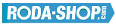# Remote ControlManufacturer Product Image Item Name Price### 61901-2RS

Thin Section Bearings Chrome Steel Rubber Seals on Both sides Dimension d=12.00 Dimension D=24.00 Dimension B=6.00 Dinamic Load Rating=2886 Static Load Rating=1466 Weight=0.01gr. Speed limit Grease=31.0 Speed limit Oil=36.0
inside diameter = 12.00
outside diameter = 24.00
width = 6.00
€4.24### 61902-2RS

Thin Section Bearings Chrome Steel Rubber Seals on Both sides Dimension d=15.00 Dimension D=28.00 Dimension B=7.00 Dinamic Load Rating=4321 Static Load Rating=2259 Weight=0.015gr. Speed limit Grease=26.0 Speed limit Oil=30.0
inside diameter = 15.00
outside diameter = 28.00
width = 7.00
€4.54### 61902-2Z

Thin Section Bearings Chrome Steel Metallic Shields on both sides Dimension d=15.00 Dimension D=28.00 Dimension B=7.00 Dinamic Load Rating=4321 Static Load Rating=2259 Weight=0.015gr. Speed limit Grease=26.0 Speed limit Oil=30.0
inside diameter = 15.00
outside diameter = 28.00
width = 7.00
€4.54### 61903

Thin Section Bearings Chrome Steel Open(No Shields) Dimension d=17.00 Dimension D=30.00 Dimension B=7.00 Dinamic Load Rating=4588 Static Load Rating=2565 Weight=0.01gr. Speed limit Grease=23.0 Speed limit Oil=38.0
inside diameter = 17.00
outside diameter = 30.00
width = 7.00
€4.54### 61903-2RS

Thin Section Bearings Chrome Steel Rubber Seals on Both sides Dimension d=17.00 Dimension D=30.00 Dimension B=7.00 Dinamic Load Rating=4588 Static Load Rating=2565 Weight=0.01gr. Speed limit Grease=23.0 Speed limit Oil=38.0
inside diameter = 17.00
outside diameter = 30.00
width = 7.00
€4.54### 61903-2Z

Thin Section Bearings Chrome Steel Metallic Shields on both sides Dimension d=17.00 Dimension D=30.00 Dimension B=7.00 Dinamic Load Rating=4588 Static Load Rating=2565 Weight=0.01gr. Speed limit Grease=23.0 Speed limit Oil=38.0
inside diameter = 17.00
outside diameter = 30.00
width = 7.00
€4.54### 61904-2RS

Thin Section Bearings Chrome Steel Rubber Seals on Both sides Dimension d=20.00 Dimension D=37.00 Dimension B=9.00 Dinamic Load Rating=6381 Static Load Rating=3682 Weight=0.04gr. Speed limit Grease=19.0 Speed limit Oil=23.0
inside diameter = 20.00
outside diameter = 37.00
width = 9.00
€4.84### 61904-2Z

Thin Section Bearings Chrome Steel Metallic Shields on both sides Dimension d=20.00 Dimension D=37.00 Dimension B=9.00 Dinamic Load Rating=6381 Static Load Rating=3682 Weight=0.04gr. Speed limit Grease=19.0 Speed limit Oil=23.0
inside diameter = 20.00
outside diameter = 37.00
width = 9.00
€4.84### 623

Miniature Metric Series Chrome Steel Open(No Shields) Dimension d=3.00 Dimension D=10.00 Dimension B=4.00 Dinamic Load Rating=631 Static Load Rating=219 Weight=0.002gr. Speed limit Grease=50.0 Speed limit Oil=60.0
inside diameter = 3.00
outside diameter = 10.00
width = 4.00
€2.24### 623-2RS

Miniature Metric Series Chrome Steel Rubber Seals on Both sides Dimension d=3.00 Dimension D=10.00 Dimension B=4.00 Dinamic Load Rating=631 Static Load Rating=219 Weight=0.002gr. Speed limit Grease=50.0 Speed limit Oil=60.0
inside diameter = 3.00
outside diameter = 10.00
width = 4.00
€2.24